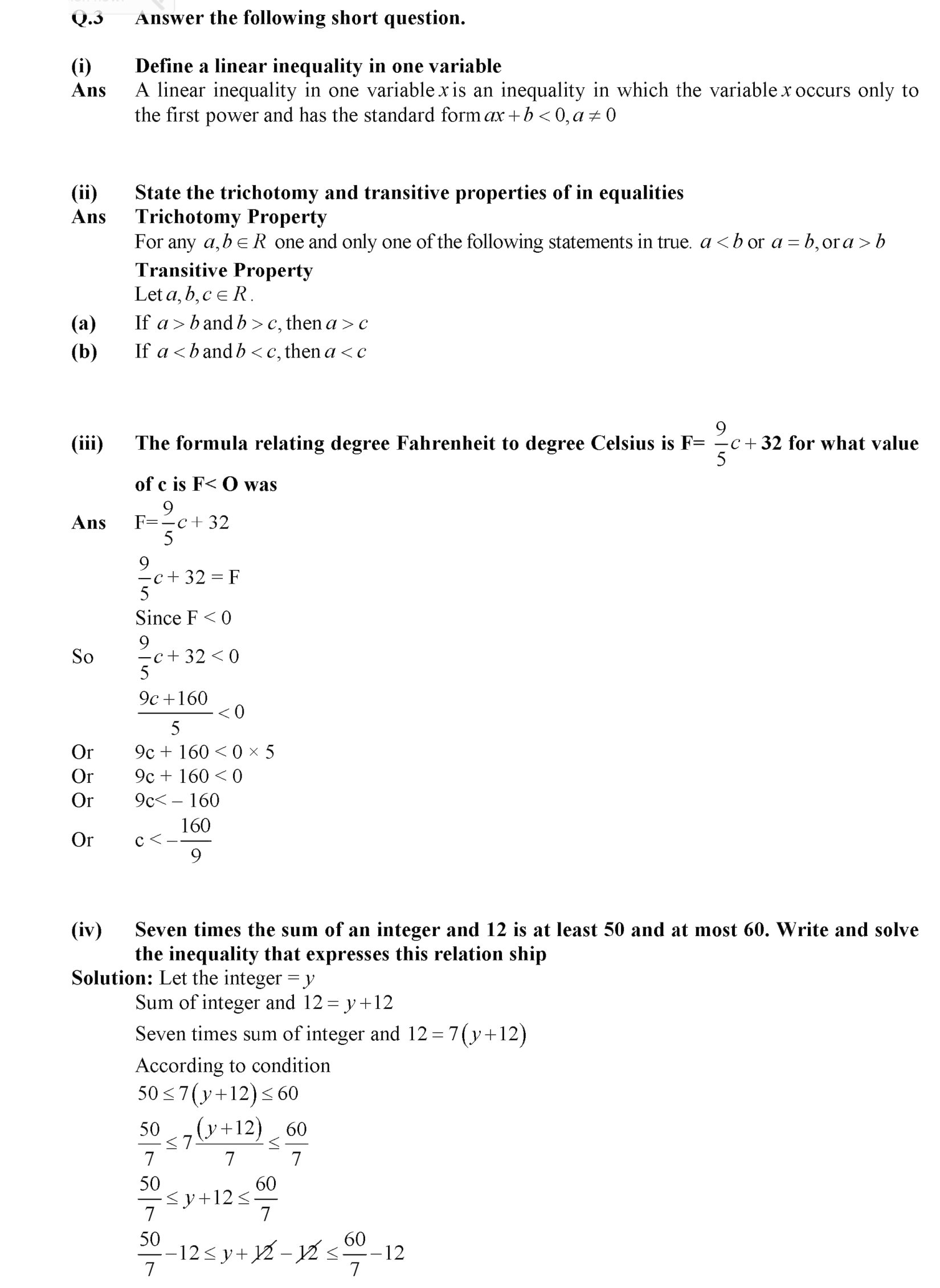# 9th class Math solved Notes Chapter Name: Linear Equations and Inequalities {Review Exercise 7}9th class Math solved Notes Chapter Name: Linear Equations and Inequalities {Review Exercise 7}

9th class Math solved Notes Chapter Name: Linear Equations and Inequalities {Review Exercise 7}